# Basics of ORP Measurement

What is ORP?

ORP stands for oxidation-reduction potential, which is a measure, in millivolts, of the tendency of a chemical substance to oxidize or reduce another chemical substance.

Oxidation

Oxidation is the loss of electrons by an atom, molecule, or ion. It may or may not be accompanied by the addition of oxygen, which is the origin of the term. Familiar examples are iron rusting and wood burning.

When a substance has been oxidized, its oxidation state increases. Many substances can exist in a number of oxidation states. A good example is sulfur, which can exhibit oxidation states of -2 (H2S); 0 (S); +4 (SO2); and +6 (SO4 -2). Substances with multiple oxidation states can be sequentially oxidized from one oxidation state to the next higher. Adjacent oxidation states of a particular substance are referred to as redox couples. In the case below, the redox couple is Fe+2/Fe: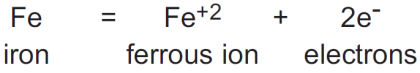The chemical equation shown above is called the half-reaction for the oxidation, because, as will be seen, the electrons lost by the iron atom cannot exist in solution and have to be accepted by another substance in solution. So the complete reaction involving the oxidation of iron will have to include another substance, which will be reduced. The oxidation reaction shown for iron is, therefore, only half of the total reaction that takes place.

Reduction

Reduction is the net gain of electrons by an atom, molecule, or ion.

When a chemical substance is reduced, its oxidation state is lowered. As was the case with oxidation, substances that can exhibit multiple oxidation states can also be sequentially reduced from one oxidation state to the next lower oxidation state.

The chemical equation shown below is the half-reaction for the reduction of chlorine: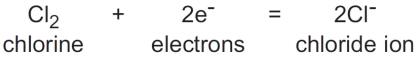The redox couple in the above case is Cl2/Cl- (chlorine/chloride).

Oxidation reactions are always accompanied by reduction reactions. The electrons lost in oxidation must have another substance as a destination, and
the electrons gained in reduction reactions have to come from a source.

When two half-reactions are combined to give the overall reaction, the electrons lost in the oxidation reaction must equal the electrons gained in the reduction reaction.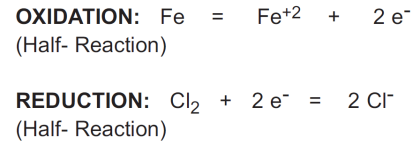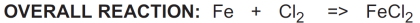In the reaction above, iron (Fe) reduces chlorine (Cl2) and is called a reductant or reducing agent.

Conversely, chlorine (Cl2) oxidizes iron (Fe) and is called an oxidant or oxidizing agent:

Oxidizing and Reducing Agents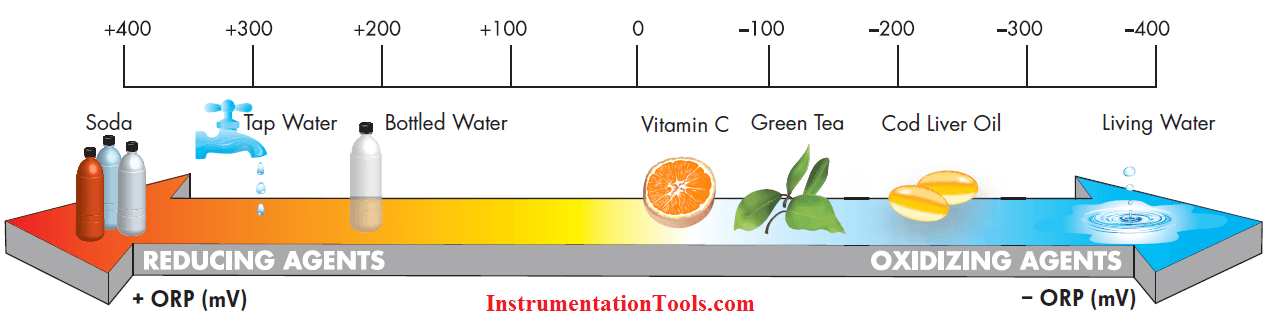Standard Potential

How easily a substance is oxidized or reduced is given by the standard potential of its redox couple, symbolized by E°. The standard potentials of quite a number of redox couples are tabulated in reference books, along with their half-reactions. All are referenced to the redox couple for hydrogen ion/hydrogen (H+/H2), which is assigned a standard potential of 0 millivolts. The standard potential refers to half reaction written as a reduction. The negative of the tabulated standard potential gives the standard potential for the oxidation half-reaction. An example of these follows: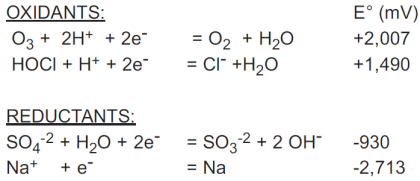ORP in Solutions

The standard potential for a half reaction is based on the assumption that the concentrations of all the chemical substances shown in the half reaction are at 1 molar concentration. In a process, however, the concentrations can vary independently of one another. So, to arrive at the ORP of a particular solution, it is necessary to use the Nernst equation to calculate the ORP for each case.

NERNST Equation for ORP

The ORP of a general half-reaction can be written in terms of molar concentrations as follows:
aA+bB+cC+ …+ ne- = xX+yY+zZ + …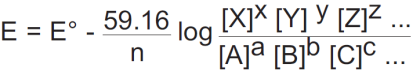Hypochlorous acid (chlorine in water) provides a useful example of the Nernst equation: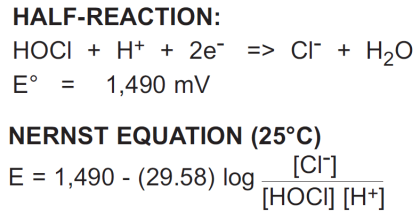Examining the hypochlorous acid/chloride equation shows some important properties of ORP:

1. The ORP depends upon the concentrations of all the substances in the half-reaction (except water). Therefore, the ORP of hypochlorous acid
depends as much on chloride ion (Cl-) and pH (H+) as it does on hypochlorous acid.

2. The ORP is a function of the logarithm of the concentration ratio.

3. The coefficient that multiplies this logarithm of concentration is equal to -59.16 mV, divided by the number of electrons in the half-reaction (n). In this case, n = 2; therefore, the coefficient is -29.58. A 10-fold change in the concentration of Cl-, HOCl, H+ will only change the ORP ±29.58mV.

4. There is no specific temperature dependence shown. Temperature can affect an ORP reaction in a variety of ways, so no general ORP temperature behavior can be characterized, as is the case with pH. Therefore, ORP measurements are almost never temperature compensated.

When checking the influence of an individual substance in the half reaction, the Nernst equation can be partitioned into individual logarithms for each substance, and the contribution of that substance calculated over its expected concentration range.

Measurement of ORP

An ORP sensor consists of an ORP electrode and a reference electrode, in much the same fashion as a pH measurement.

ORP Electrode

The principle behind the ORP measurement is the use of an inert metal electrode (platinum, sometimes gold), which, due to its low resistance, will give up electrons to an oxidant or accept electrons from a reductant. The ORP electrode will continue to accept or give up electrons until it develops a potential, due to the build up charge, which is equal to the ORP of the solution. The typical accuracy of an ORP measurement is ±5 mV.

Sometimes the exchange of electrons between the ORP electrode and certain chemical substances is hampered by a low rate of electron exchange (exchange current density). In these cases, ORP may respond more strongly to a second redox couple in the solution (like dissolved oxygen). This leads to measurement errors, and it is recommended that new ORP applications be checked out in the laboratory before going on-line.

Reference Electrode

The reference electrode used for ORP measurements is typically the same silver-silver chloride electrode used with pH measurements. In contrast with pH measurements, some offset in the reference is tolerable in ORP since, as will be seen, the mV changes measured in most ORP applications are large. In certain specific applications (for example, bleach production), an ORP sensor may use a silver billet as a reference, or even a pH electrode.Applications

Due to its dependence upon the concentrations of multiple chemical substances, the application of ORP for many has been a puzzling and often frustrating experience. When considering ORP for a particular application, it is necessary to know the half-reaction involved and the concentration range of all the substances appearing in the half-reaction. It is also necessary to use the Nernst equation to get an idea of the expected ORP behavior.

Concentration Measurement with ORP

ORP is often applied to a concentration measurement (chlorine in water for example) without a clear understanding of all the factors involved. When the equation for the ORP of a hypochlorous solution (in the previous section) is considered, the problems associated with a concentration measurement can be outlined:

1. The ORP depends upon chloride ion (Cl-) and pH (H+) as much as it does hypochlorous acid (chlorine in water). Any change in the chloride concentration or pH will affect the ORP. Therefore, to measure chlorine accurately, chloride ion and pH must be measured to a high accuracy or carefully controlled to constant values.

2. To calculate hypochlorous concentration from the measured millivolts, the measured millivolts will appear as the exponent of 10. The typical accuracy of an ORP measurement is ±5 mV. This error alone will result in the calculated hypochlorous acid concentration being off by more than ±30%. Any drift in the reference electrode or the ORP analyzer will only add to this error.

3. Any change in the ORP with temperature is not compensated, further increasing the error in the derived concentration.

In general, ORP is not a good technique to apply to concentration measurements. Virtually all ORP halfreactions involve more than one substance, and the vast majority have pH dependence. The logarithmic dependence of ORP on concentration multiplies any errors in the measured millivolts.

ORP Applications

Applications that use ORP for monitoring and controlling oxidation-reduction reactions include cyanide destruction, dechlorination, chromate reduction, hypochlorite bleach production, and chlorine and chlorine dioxide scrubber monitoring using bisulfite.

Concentration measurement with ORP, as was seen, is problematic, but ORP can be used in some cases for leak detection to detect the presence of an oxidant or reductant.

Finally, ORP is measured, in some instances, for the control of biological growth. The principle behind these applications is that a minimum ORP value will successfully destroy microorganisms. This approach has been used in the chlorination of swimming pools and cooling towers. It should be noted that both of these applications also include pH control.

Conclusion

The oxidation-reduction potential of a solution is a measure of the oxidizing or reducing power of the solution. Every oxidation or reduction can be characterized by a half-reaction, which gives all of the chemical substances participating in the reaction. The ORP of the solution depends upon the logarithm of the concentrations of the substances participating in the halfreaction. The ORP can be calculated using the Nernst equation. ORP is not a good method for measuring concentration due to its logarithmic dependence on concentration and its dependence on multiple solution components. The best use of an ORP measurement is in monitoring and controlling oxidation-reduction reactions.